# Setup for 4 Player a Game of Food Chain Magnate

Originally generated on 1/11/2019 7:21:37 AM
A direct link to this setup is http://www.VentersConsulting.com/BoardgameHelpers/FoodChainMagnateGenerator.aspx?LoadGame=\$2a\$04\$wa6LQZ9LyCA5yu8uaM3Z1O

## Player Setup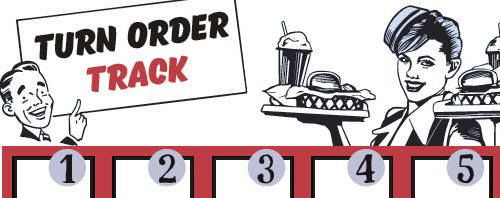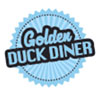## Other Setup

Bank starts with \$200.00
Remove these Billboards: #16
Number of 1x Employee cards used = 2

## Map (Random)

Click here to view Map Tile Lettering Key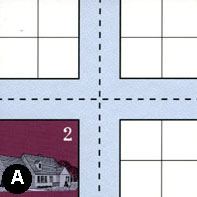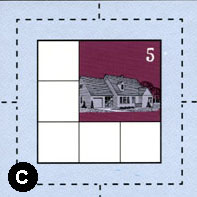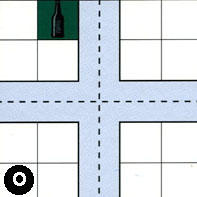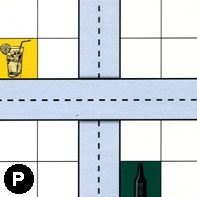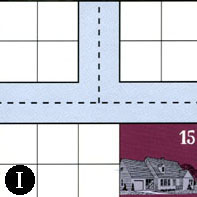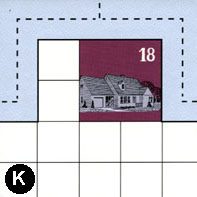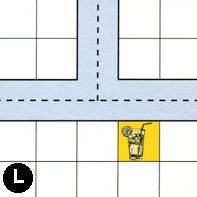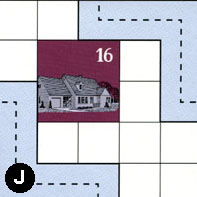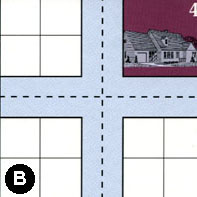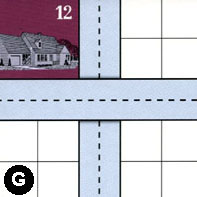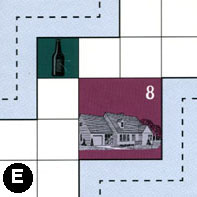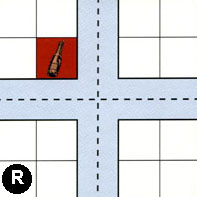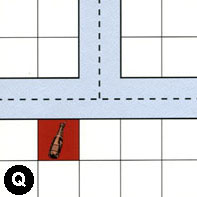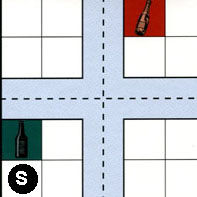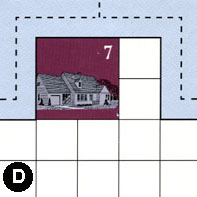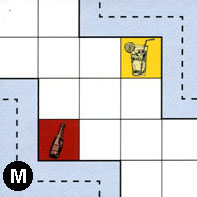### Map Stats

Map Option = Random
Total Number of Tiles = 16
Total Number of Starting Houses = 9
Total Number of Beer Spots = 4
Total Number of Soda Spots = 4
Total Number of Lemonade Spots = 3
Total Number of Drink Spots = 11
Number of independent path systems = 3
Number of independent neighborhoods = 18

Path System 1
Size = Large
Number of Tiles = 16
Number of Paths = 58
Contains Loop(s) = No
Number of Starting Houses = 10
Starting Houses Pct of Map (%) = 111
Number of Beer Spots = 4
Number of Soda Spots = 4
Number of Lemonade Spots = 1
Number of Drink Spots = 9
Drink Spots Pct of Map (%) = 82
Tile Ids = A, B, C, D, E, G, I, J, K, L, M, O, P, Q, R, S
Paths = AWE, IWN, IWE, INE, AWN, ANE, AES, CWE, OWS, OWN, PNS, ONE, LWE, EES, RWN, MWS, RWE, JWS, RNE, RNS, RWS, RES, LWN, KWN, CWN, ONS, OES, LNE, KNE, GWE, SWS, DWN, DWE, EWN, GNS, BWE, BWN, BNE, BES, QWN, QWE, SWE, DNE, SWN, SNE, QNE, BWS, BNS, SES, SNS, KWE, CNE, AWS, ANS, CES, CWS, CNS, OWE What do these mean?
Starting House Ids = 0, 2, 4, 5, 7, 8, 12, 15, 16, 18
Path System 2
Size = Small
Number of Tiles = 2
Number of Paths = 2
Contains Loop(s) = No
Number of Starting Houses = 2
Starting Houses Pct of Map (%) = 22
Number of Beer Spots = 0
Number of Soda Spots = 0
Number of Lemonade Spots = 1
Number of Drink Spots = 1
Drink Spots Pct of Map (%) = 9
Tile Ids = J, P
Paths = PWE, JEN What do these mean?
Starting House Ids = 0, 16
Path System 3
Size = Tiny
Number of Tiles = 1
Number of Paths = 1
Contains Loop(s) = No
Number of Starting Houses = 1
Starting Houses Pct of Map (%) = 11
Number of Beer Spots = 0
Number of Soda Spots = 0
Number of Lemonade Spots = 1
Number of Drink Spots = 1
Drink Spots Pct of Map (%) = 9
Tile Ids = M
Paths = MEN What do these mean?
Starting House Ids = 0

Neighborhood 1
Total Size = Small
Number of Total Spaces = 4
Number of Empty Spaces (for new houses & gardens) = 4
Number of Beer Spots = 0
Number of Soda Spots = 0
Number of Lemonade Spots = 0
Number of Drink Spots = 0
Number of Starting Houses = 0
Starting Houses Pct of Map (%) = 0
Tile Ids = A
Neighborhood 2
Total Size = Small
Number of Total Spaces = 4
Number of Empty Spaces (for new houses & gardens) = 4
Number of Beer Spots = 0
Number of Soda Spots = 0
Number of Lemonade Spots = 0
Number of Drink Spots = 0
Number of Starting Houses = 0
Starting Houses Pct of Map (%) = 0
Tile Ids = A
Neighborhood 3
Total Size = Small
Number of Total Spaces = 4
Number of Empty Spaces (for new houses & gardens) = 4
Number of Beer Spots = 0
Number of Soda Spots = 0
Number of Lemonade Spots = 0
Number of Drink Spots = 0
Number of Starting Houses = 0
Starting Houses Pct of Map (%) = 0
Tile Ids = O
Neighborhood 4
Total Size = Medium
Number of Total Spaces = 8
Number of Empty Spaces (for new houses & gardens) = 7
Number of Beer Spots = 1
Number of Soda Spots = 0
Number of Lemonade Spots = 0
Number of Drink Spots = 1
Number of Starting Houses = 0
Starting Houses Pct of Map (%) = 0
Tile Ids = O, P
Neighborhood 5
Total Size = Small
Number of Total Spaces = 4
Number of Empty Spaces (for new houses & gardens) = 3
Number of Beer Spots = 1
Number of Soda Spots = 0
Number of Lemonade Spots = 0
Number of Drink Spots = 1
Number of Starting Houses = 0
Starting Houses Pct of Map (%) = 0
Tile Ids = P
Neighborhood 6
Total Size = Medium
Number of Total Spaces = 9
Number of Empty Spaces (for new houses & gardens) = 5
Number of Beer Spots = 0
Number of Soda Spots = 0
Number of Lemonade Spots = 0
Number of Drink Spots = 0
Number of Starting Houses = 1
Starting Houses Pct of Map (%) = 11
Tile Ids = C
Starting House Ids = 5
Neighborhood 7
Total Size = Medium
Number of Total Spaces = 8
Number of Empty Spaces (for new houses & gardens) = 8
Number of Beer Spots = 0
Number of Soda Spots = 0
Number of Lemonade Spots = 0
Number of Drink Spots = 0
Number of Starting Houses = 0
Starting Houses Pct of Map (%) = 0
Tile Ids = A, I
Neighborhood 8
Total Size = Large
Number of Total Spaces = 46
Number of Empty Spaces (for new houses & gardens) = 30
Number of Beer Spots = 0
Number of Soda Spots = 0
Number of Lemonade Spots = 0
Number of Drink Spots = 0
Number of Starting Houses = 4
Starting Houses Pct of Map (%) = 44
Tile Ids = A, B, G, I, K
Starting House Ids = 2, 4, 15, 18
Neighborhood 9
Total Size = Medium
Number of Total Spaces = 8
Number of Empty Spaces (for new houses & gardens) = 8
Number of Beer Spots = 0
Number of Soda Spots = 0
Number of Lemonade Spots = 0
Number of Drink Spots = 0
Number of Starting Houses = 0
Starting Houses Pct of Map (%) = 0
Tile Ids = L, O
Neighborhood 10
Total Size = Large
Number of Total Spaces = 41
Number of Empty Spaces (for new houses & gardens) = 35
Number of Beer Spots = 0
Number of Soda Spots = 0
Number of Lemonade Spots = 2
Number of Drink Spots = 2
Number of Starting Houses = 1
Starting Houses Pct of Map (%) = 11
Tile Ids = J, L, O, P, R
Starting House Ids = 16
Neighborhood 11
Total Size = Small
Number of Total Spaces = 4
Number of Empty Spaces (for new houses & gardens) = 4
Number of Beer Spots = 0
Number of Soda Spots = 0
Number of Lemonade Spots = 0
Number of Drink Spots = 0
Number of Starting Houses = 0
Starting Houses Pct of Map (%) = 0
Tile Ids = P
Neighborhood 12
Total Size = Large
Number of Total Spaces = 58
Number of Empty Spaces (for new houses & gardens) = 42
Number of Beer Spots = 1
Number of Soda Spots = 2
Number of Lemonade Spots = 1
Number of Drink Spots = 4
Number of Starting Houses = 3
Starting Houses Pct of Map (%) = 33
Tile Ids = D, E, G, L, M, R
Starting House Ids = 7, 8, 12
Neighborhood 13
Total Size = Medium
Number of Total Spaces = 8
Number of Empty Spaces (for new houses & gardens) = 8
Number of Beer Spots = 0
Number of Soda Spots = 0
Number of Lemonade Spots = 0
Number of Drink Spots = 0
Number of Starting Houses = 0
Starting Houses Pct of Map (%) = 0
Tile Ids = B, Q
Neighborhood 14
Total Size = Medium
Number of Total Spaces = 16
Number of Empty Spaces (for new houses & gardens) = 16
Number of Beer Spots = 0
Number of Soda Spots = 0
Number of Lemonade Spots = 0
Number of Drink Spots = 0
Number of Starting Houses = 0
Starting Houses Pct of Map (%) = 0
Tile Ids = B, G, Q, S
Neighborhood 15
Total Size = Medium
Number of Total Spaces = 8
Number of Empty Spaces (for new houses & gardens) = 7
Number of Beer Spots = 1
Number of Soda Spots = 0
Number of Lemonade Spots = 0
Number of Drink Spots = 1
Number of Starting Houses = 0
Starting Houses Pct of Map (%) = 0
Tile Ids = G, S
Neighborhood 16
Total Size = Small
Number of Total Spaces = 4
Number of Empty Spaces (for new houses & gardens) = 4
Number of Beer Spots = 0
Number of Soda Spots = 0
Number of Lemonade Spots = 0
Number of Drink Spots = 0
Number of Starting Houses = 0
Starting Houses Pct of Map (%) = 0
Tile Ids = R
Neighborhood 17
Total Size = Medium
Number of Total Spaces = 14
Number of Empty Spaces (for new houses & gardens) = 12
Number of Beer Spots = 0
Number of Soda Spots = 2
Number of Lemonade Spots = 0
Number of Drink Spots = 2
Number of Starting Houses = 0
Starting Houses Pct of Map (%) = 0
Tile Ids = Q, S
Neighborhood 18
Total Size = Small
Number of Total Spaces = 4
Number of Empty Spaces (for new houses & gardens) = 4
Number of Beer Spots = 0
Number of Soda Spots = 0
Number of Lemonade Spots = 0
Number of Drink Spots = 0
Number of Starting Houses = 0
Starting Houses Pct of Map (%) = 0
Tile Ids = S

v8.3.2